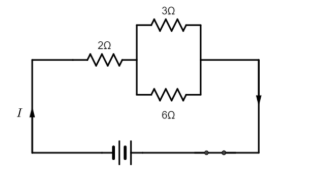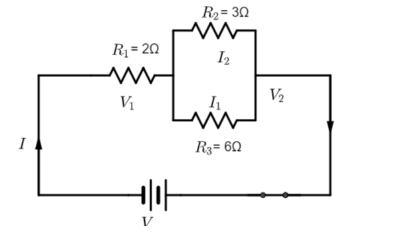QuestionAnswers

# In the given electric circuit, the current flowing through the 3ohm resistor is 1ampere. Find the voltage of the battery and the circuit drawn from it.Hint: In a parallel combination of resistors, the current divides but voltage between the terminals is the same and reciprocal of the total resistance is the sum of the reciprocal of individual resistances.
In series combination of resistors voltage divides between two resistors but same current flows through them and effective resistance is the sum of the two resistances.

Consider circuit diagram, where ${{R}_{1}}=2\Omega ,{{R}_{2}}=3\Omega$ and ${{R}_{3}}=6\Omega$. Let ${{I}_{2}}=1A$ is the current flowing through ${{R}_{2}}$ as given in the question.Now we have to find I and V which are total current and potential respectively.
Now first part to find total current I, we have,
$I={{I}_{1}}+{{I}_{2}}$ …….. (1)
we already know ${{I}_{2}}$ as it is given in question we have to find ${{I}_{1}}$
To find ${{I}_{1}}$ :
$I{}_{1}=\dfrac{{{V}_{2}}}{{{R}_{3}}}$ ….. (2)
the same voltage ${{V}_{2}}$ flows through ${{R}_{3}}$ as they are aligned parallel to each other.
but we don’t know ${{V}_{2}}$, it can be determined by using the formula:
${{V}_{2}}={{I}_{2}}{{R}_{2}}$ (Ohm’s law)
on substituting values of resistance and current we get,
${{V}_{2}}=1\times 3=3V$
On substituting ${{V}_{2}}$ in (2) we get
\begin{align} & {{I}_{1}}=\dfrac{{{V}_{2}}}{{{R}_{3}}}=\dfrac{3}{6} \\ & {{I}_{1}}=\dfrac{1}{2}A=0.5A \\ \end{align}
Now we know both ${{I}_{1}}$and${{I}_{2}}$ substituting in (1) we get
\begin{align} & I=1+0.5 \\ & I=1.5A \\ \end{align}
Thus, total current flowing through the circuit is found.
Now second part to find total potential V, we have,
$V={{V}_{1}}+{{V}_{2}}$ …… (3)
We know what is ${{V}_{2}}$ but have to find ${{V}_{1}}$ ,
${{V}_{1}}=I{{R}_{1}}$
on substituting I and ${{R}_{1}}$ we get
\begin{align} & {{V}_{1}}=1.5\times 2 \\ & {{V}_{1}}=3V \\ \end{align}
on substituting values in (3)
\begin{align} & V=3+3 \\ & V=6V \\ \end{align}
Thus, total potential of the circuit is found.

Note: Students should remember all the above formulas to solve this type of question.
Students may get confused about V and I which is constant, and which will vary when connected in series and parallel. Always remember that in series combination of resistors voltage divides current remains same between resistors and in parallel combination, the current divides but voltage remains constant.
View Notes
Electric Current in ConductorsElectric Current and Its EffectsChemical Effects of Electric CurrentMagnetic Effects of Electric CurrentIntegrated CircuitElectric CarElectric PowerCBSE Class 12 Physics Electric Charges and Fields FormulaElectric Potential - Point ChargeElectric Charge and Static ElectricityCBSE Class 8 Science Chemical Effects of Electric Current WorksheetsCBSE Class 7 Science Electric Current and Its Effects WorksheetsImportant Questions for CBSE Class 12 Physics Chapter 1 - Electric Charges and FieldsImportant Questions for CBSE Class 12 Physics Chapter 3 - Current ElectricityImportant Questions for CBSE Class 8 Science Chapter 14 - Chemical Effects of Electric CurrentImportant Questions for CBSE Class 12 Physics Chapter 7 - Alternating CurrentImportant Questions for CBSE Class 7 Science Chapter 14 - Electric Current and Its EffectsImportant Questions for CBSE Class 10 Science Chapter 13 - Magnetic Effects of Electric CurrentNCERT Books Free Download for Class 12 Physics Chapter 1 - Electric Charges and FieldsImportant Questions for CBSE Class 6 Social Science The Earth Our Habitat Chapter 1 - The Earth In The Solar SystemCBSE Class 12 Physics Question Paper 2020Previous Year Question Paper for CBSE Class 12 PhysicsPrevious Year Question Paper for CBSE Class 12 Physics - 2015Previous Year Question Paper for CBSE Class 12 Physics - 2018Previous Year Question Paper for CBSE Class 12 Physics - 2014Previous Year Question Paper for CBSE Class 12 Physics - 2013Previous Year Question Paper for CBSE Class 12 Physics - 2019Previous Year Physics Question Paper for CBSE Class 12 - 2017Physics Question Paper for CBSE Class 12 - 2016 Set 1 CPrevious Year Question Paper for CBSE Class 12 Physics - 2016 Set 1 SHC Verma Class 12 Physics Part-2 Solutions for Chapter 32 - Electric Current in ConductorsNCERT Solutions for Class 10 Science Chapter 13Electric Current and Its Effects NCERT Solutions - Class 7 ScienceChemical Effects of Electric Current NCERT Solutions - Class 8 ScienceNCERT Solutions for Class 12 Physics Chapter 1Lakhmir Singh Physics Class 10 Solutions Chapter 2 - Magnetic Effects of Electric CurrentHC Verma Class 12 Physics Part-2 Solutions for Chapter 29 - Electric Field and PotentialNCERT Solutions for Class 12 Physics Chapter 1 Electric Charges and Fields In HindiNCERT Solutions for Class 12 Physics Chapter 7NCERT Solutions for Class 12 Physics Chapter 3